# NCERT Solutions for Class 9 Maths Chapter 10 Exercise 10.5 – Circles

Download NCERT Solutions for Class 9 Maths Chapter 10 Exercise 10.5 – Circles. This Exercise contains 12 questions, for which detailed answers have been provided in this note. In case you are looking at studying the remaining Exercise for Class 9 for Maths NCERT solutions for other Chapters, you can click the link at the end of this Note.

### NCERT Solutions for Class 9 Maths Chapter 10 Exercise 10.5 – Circles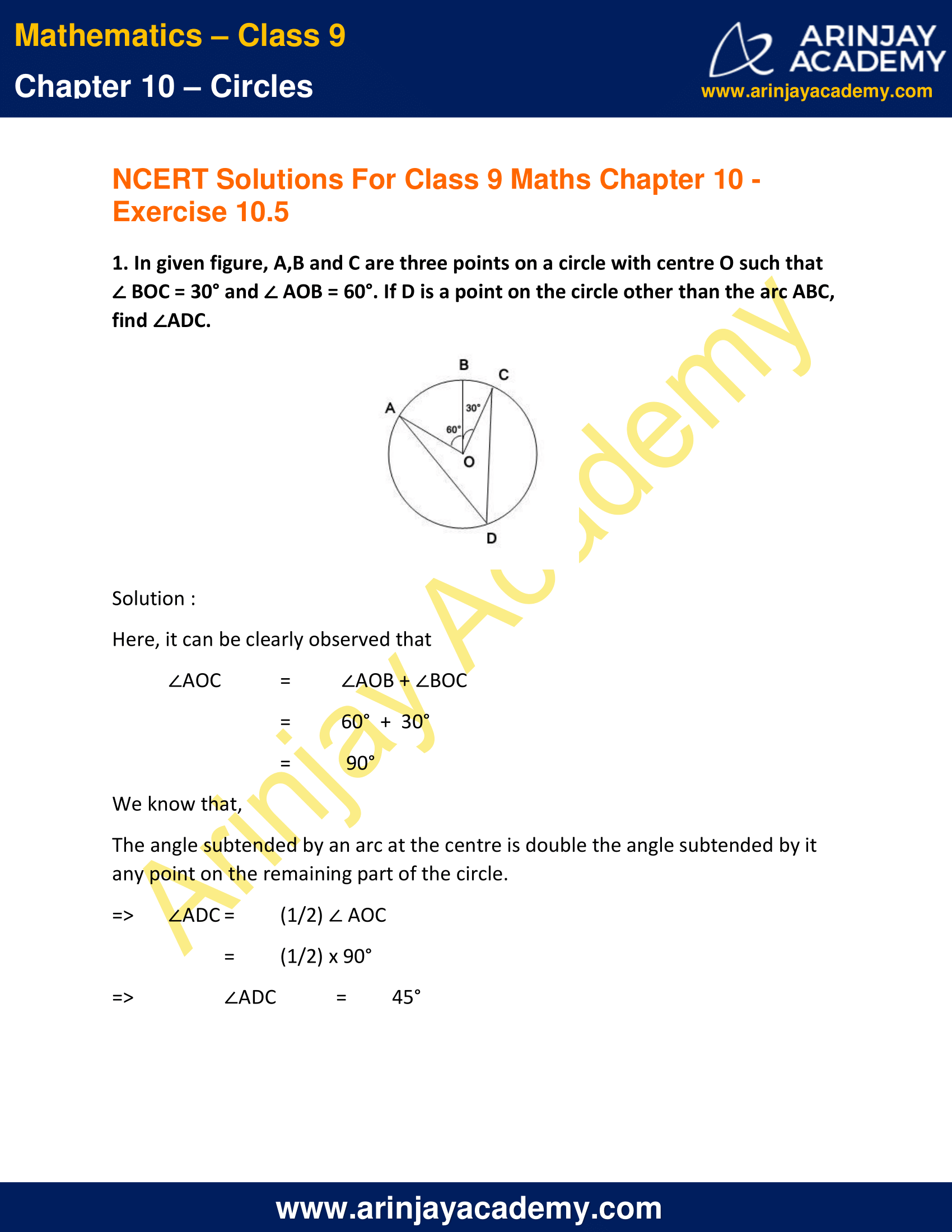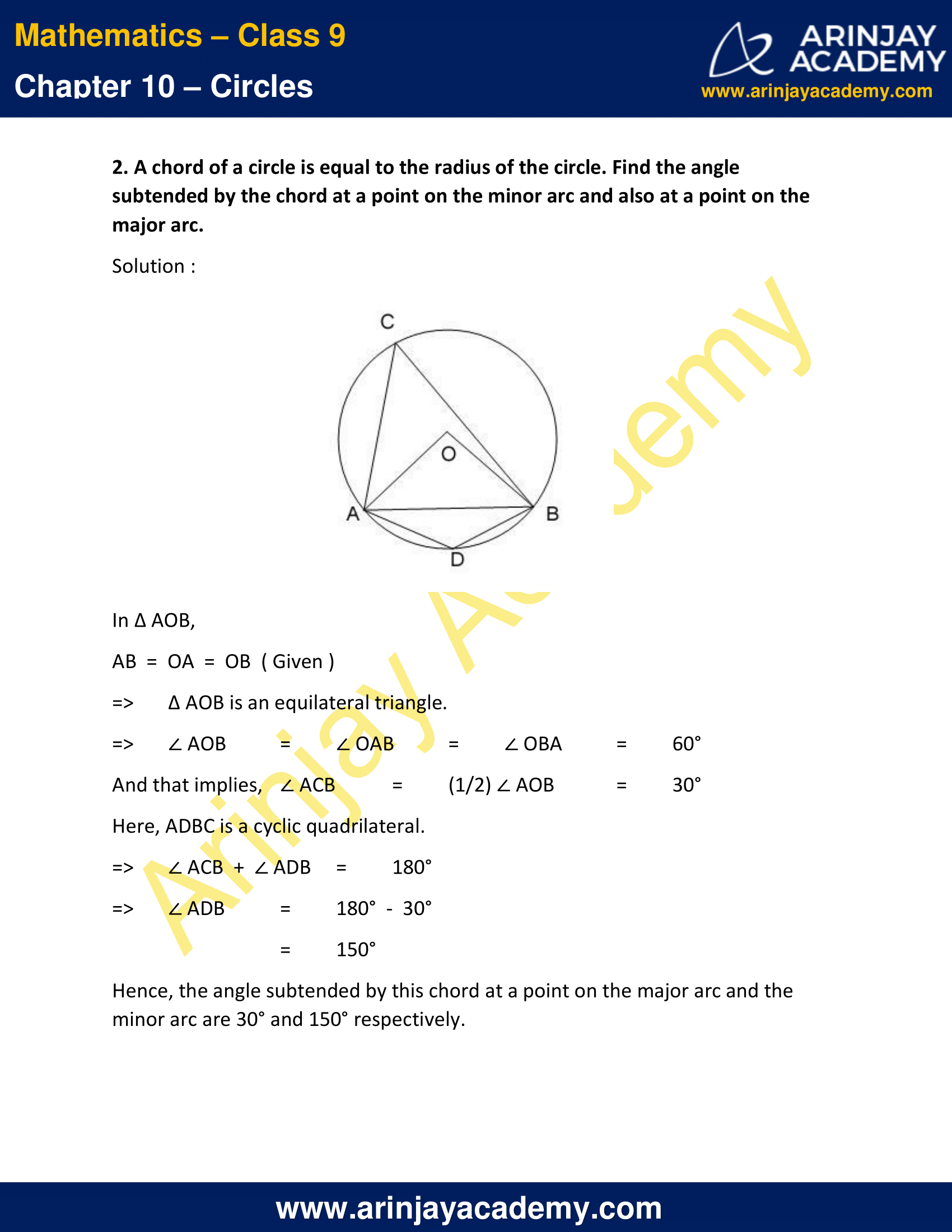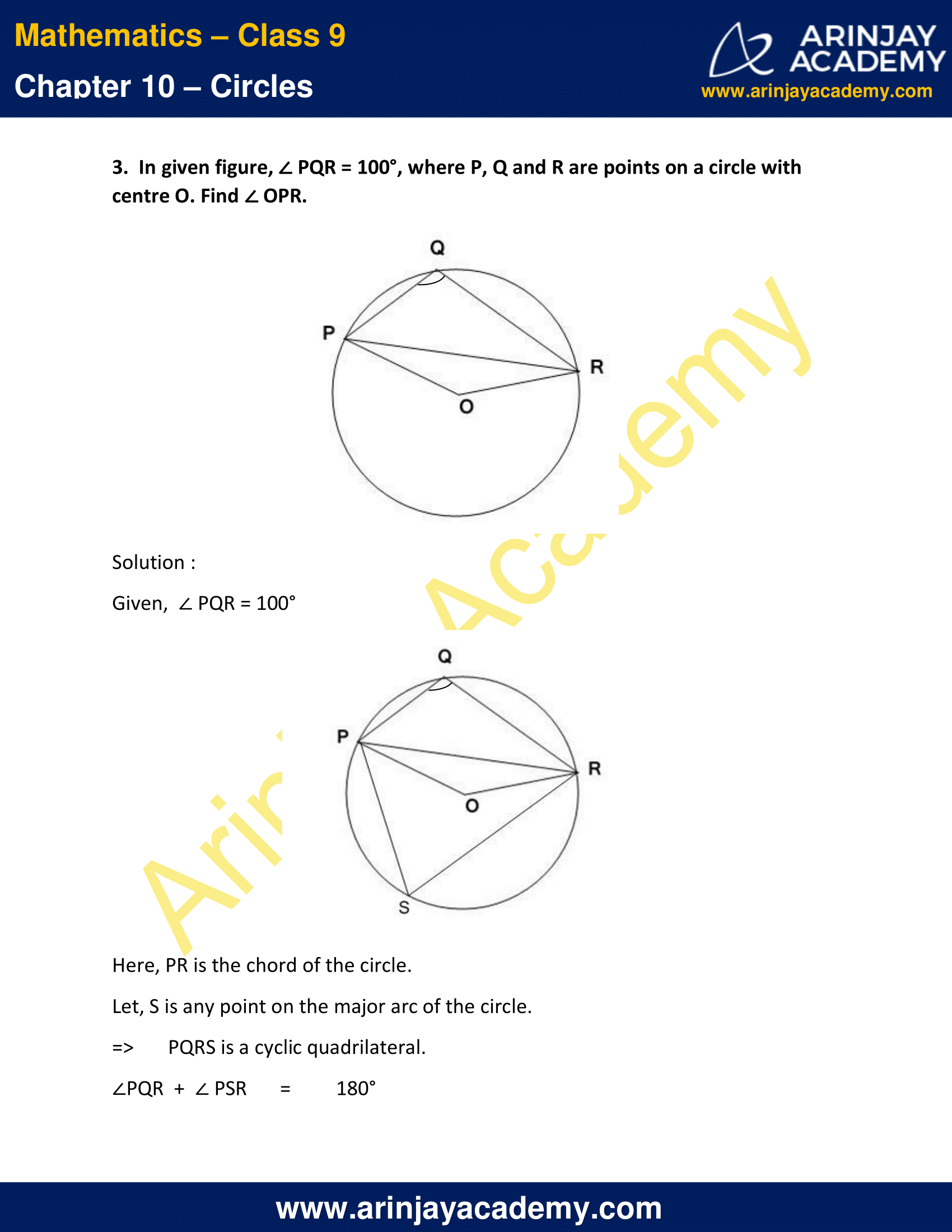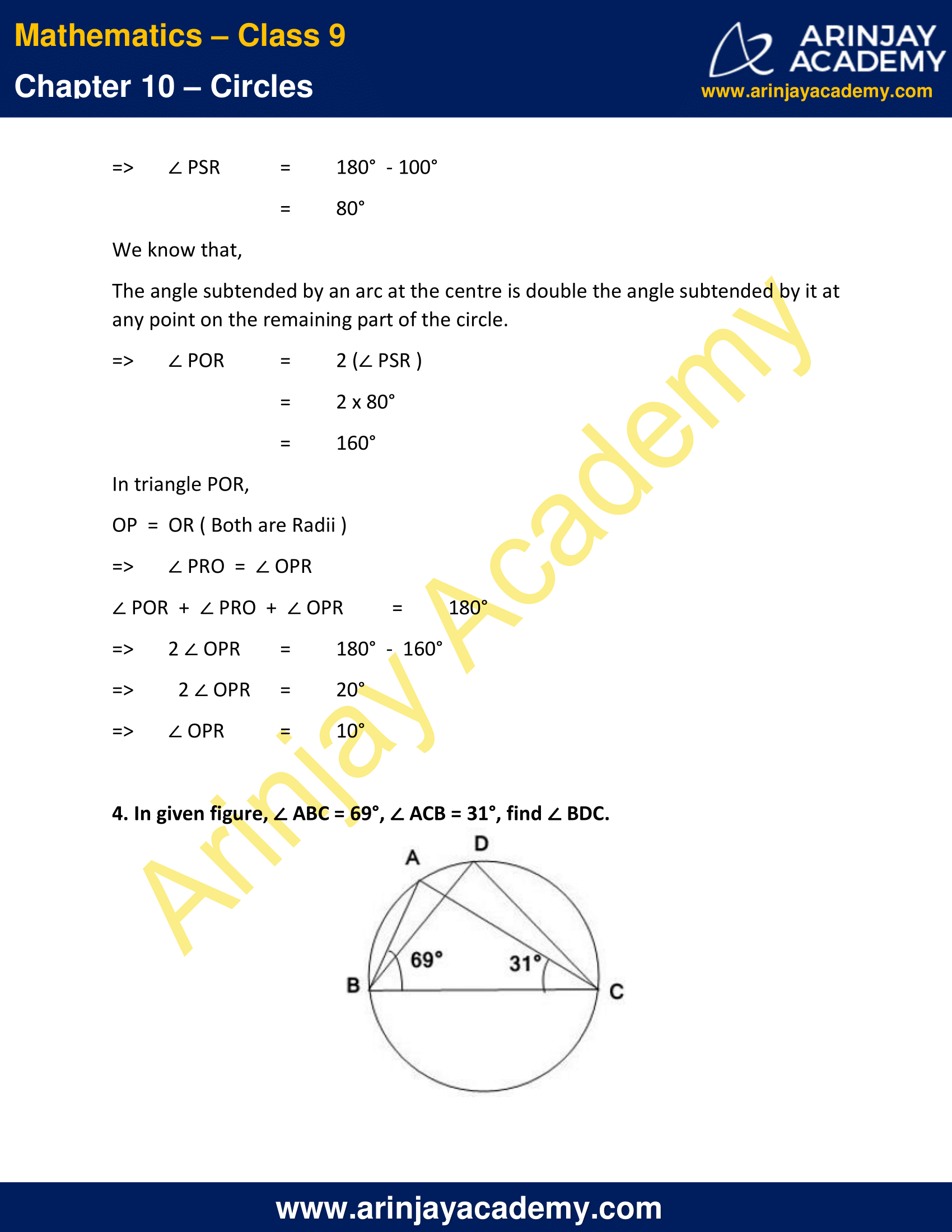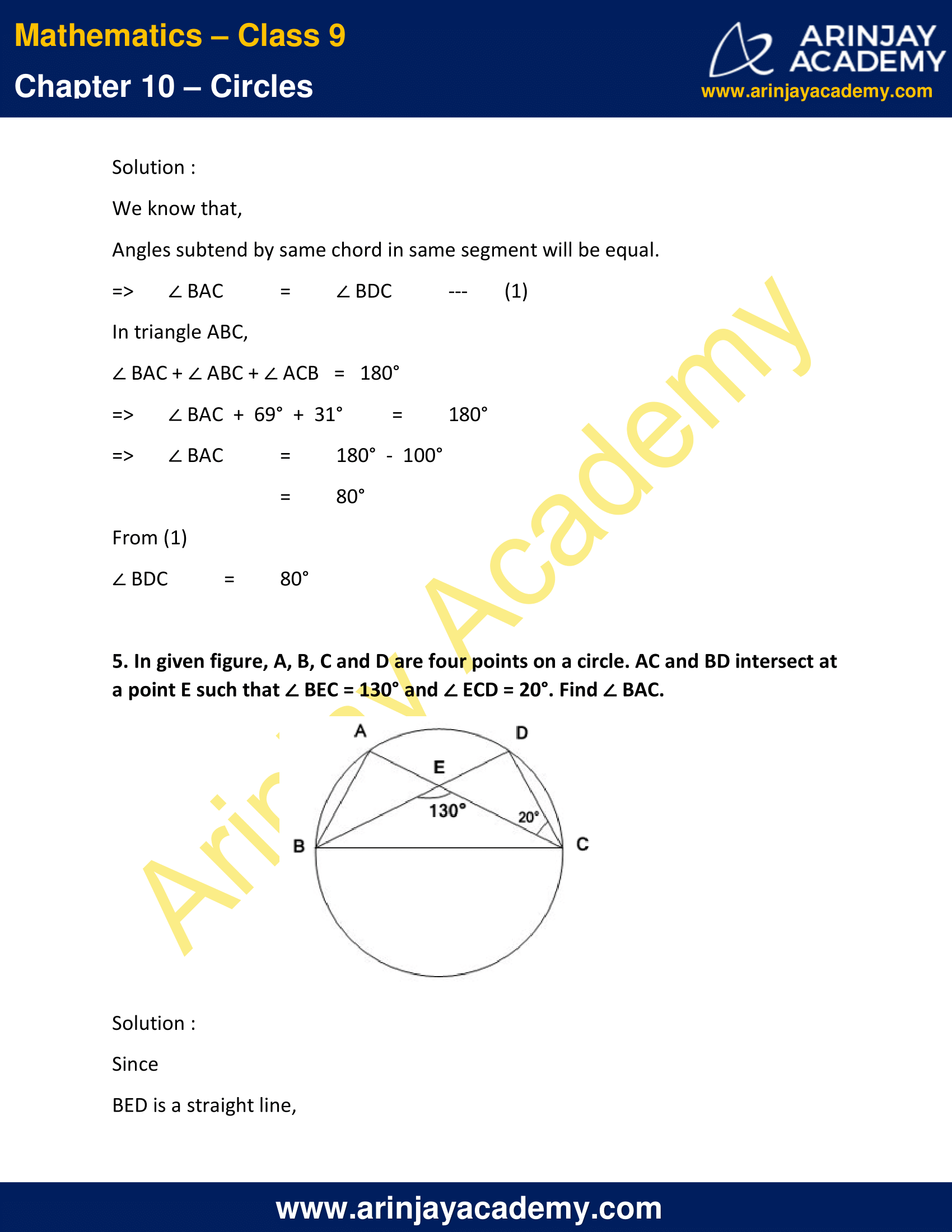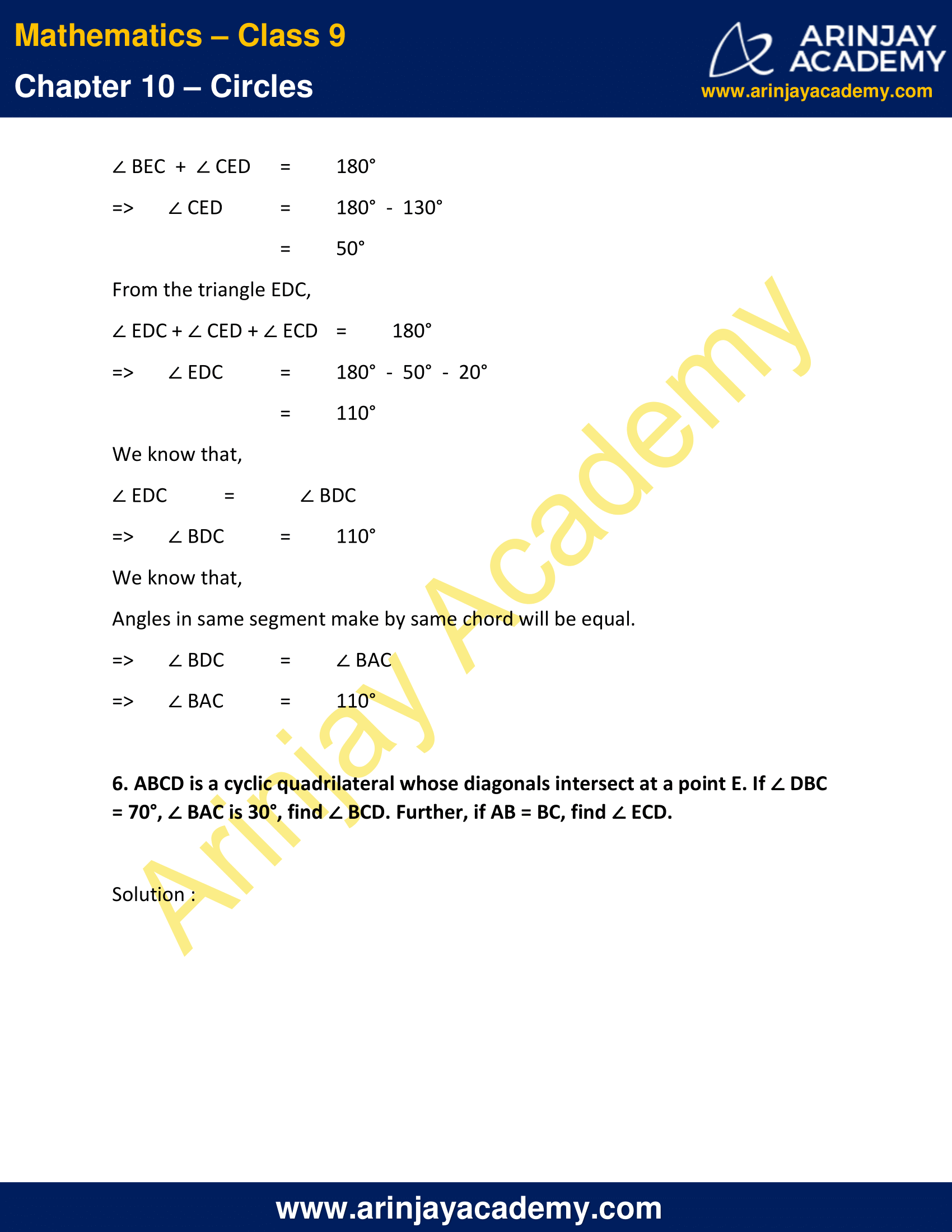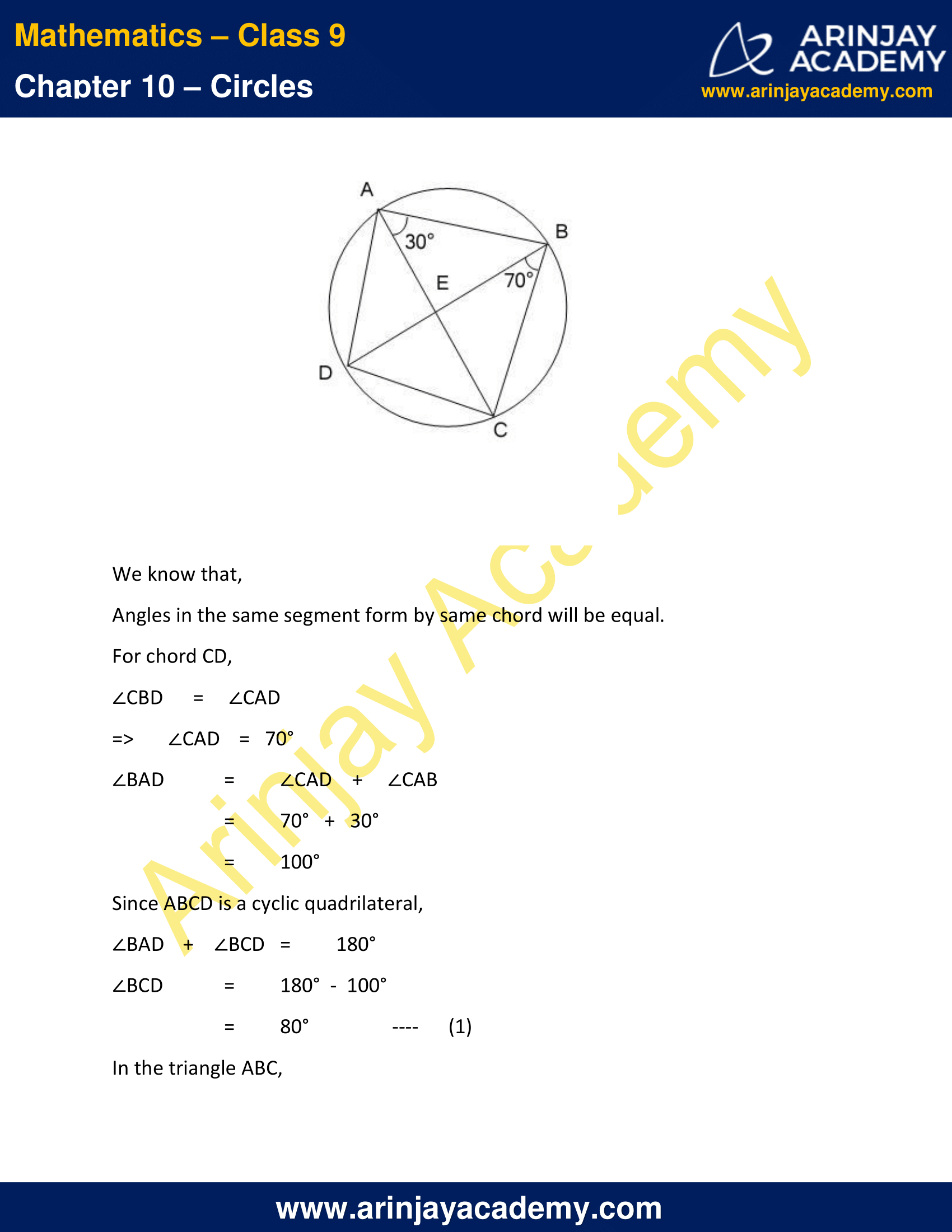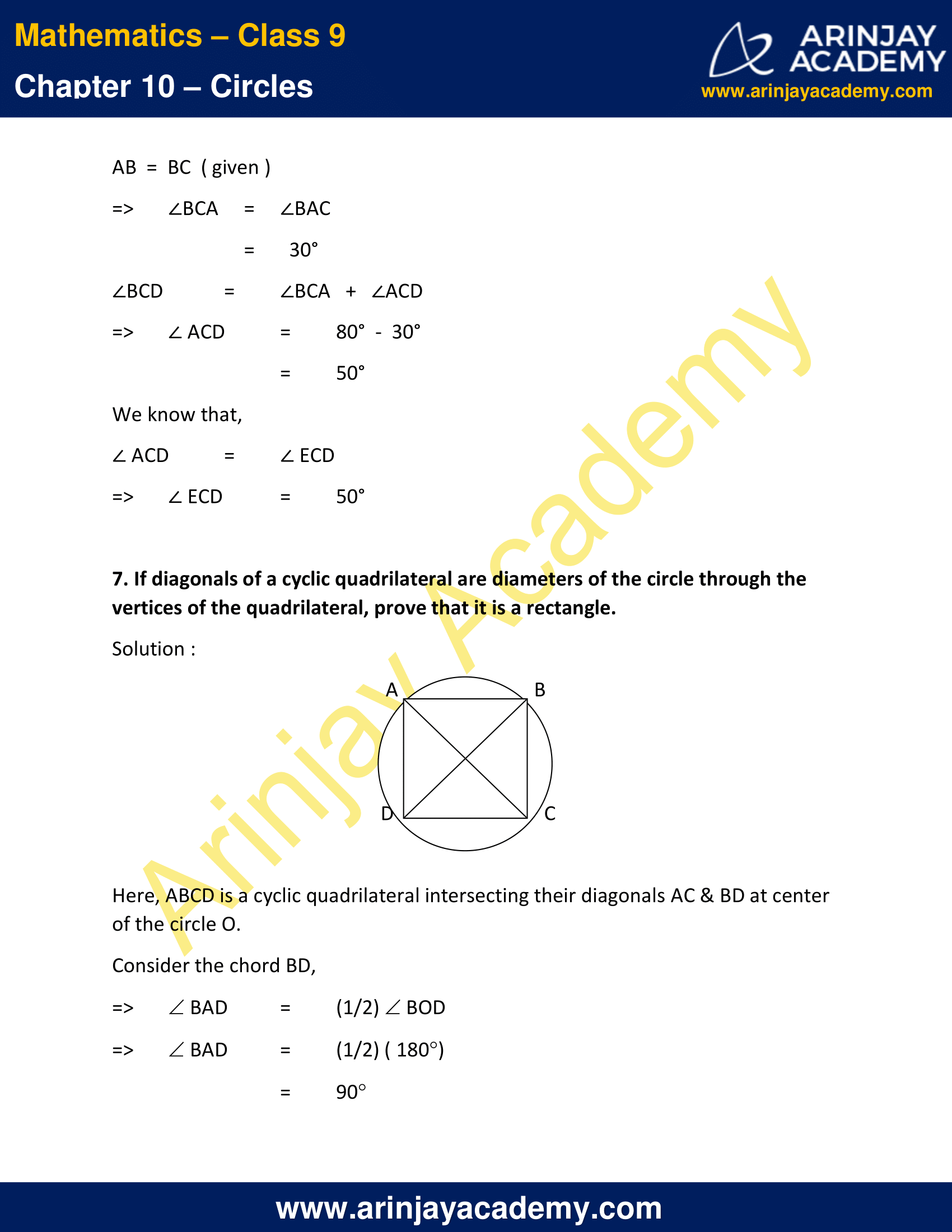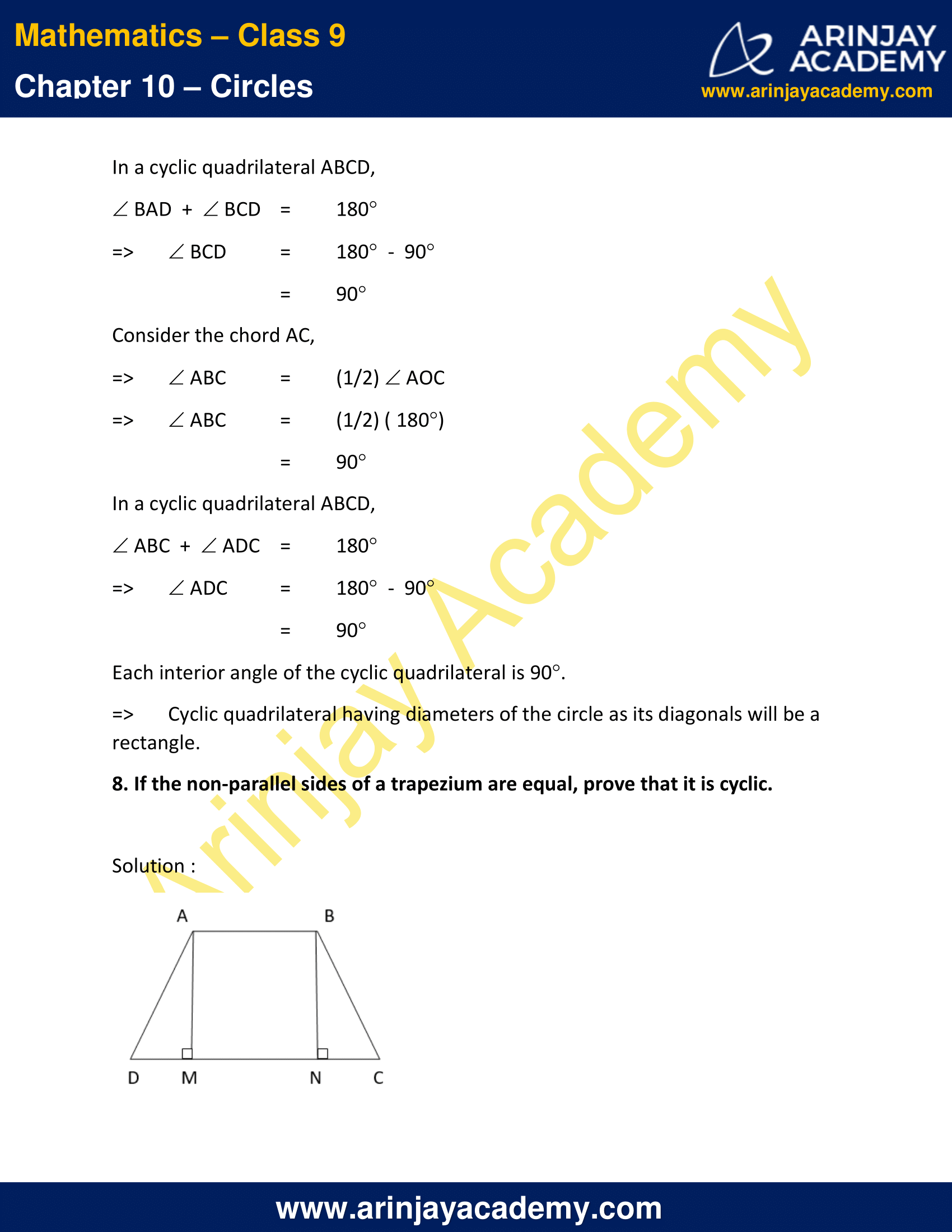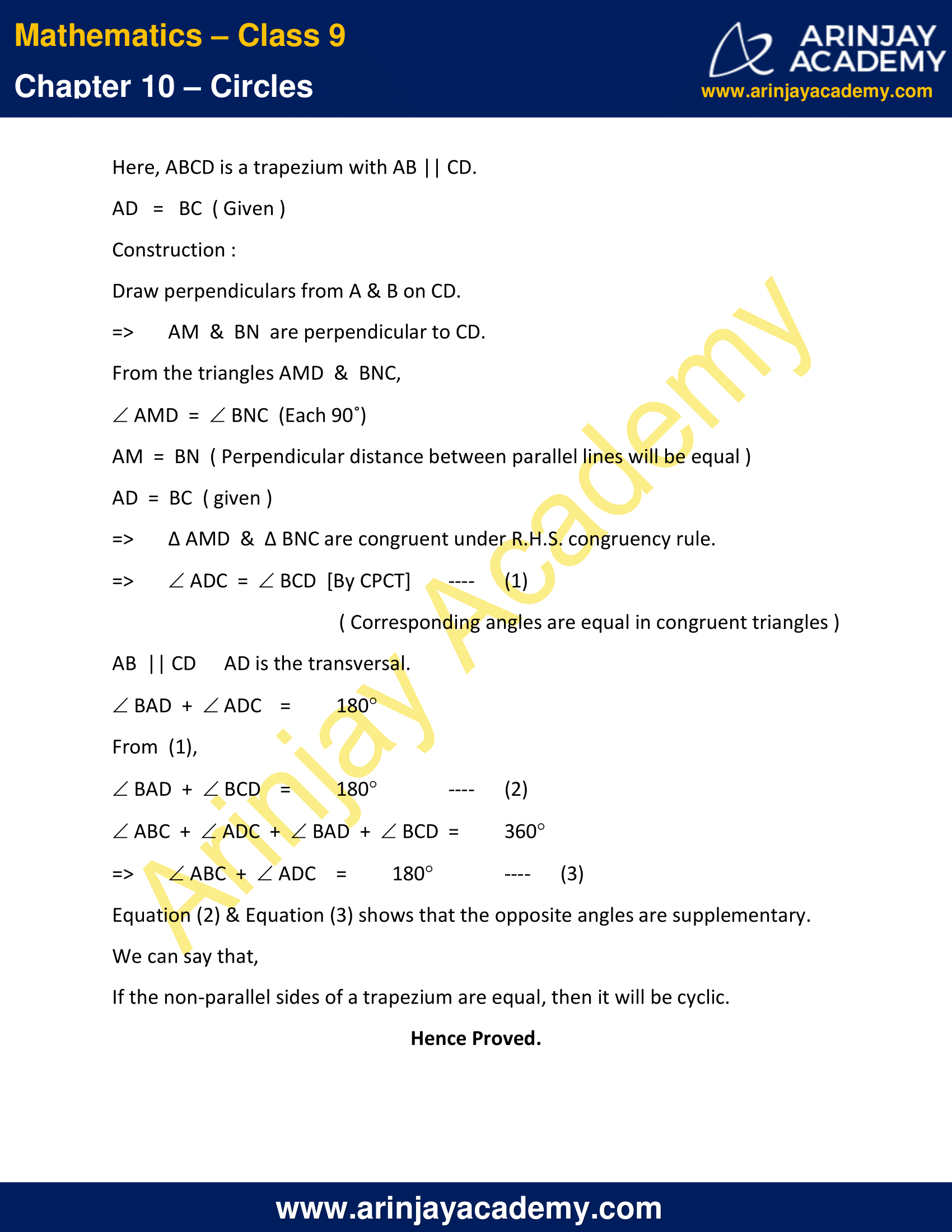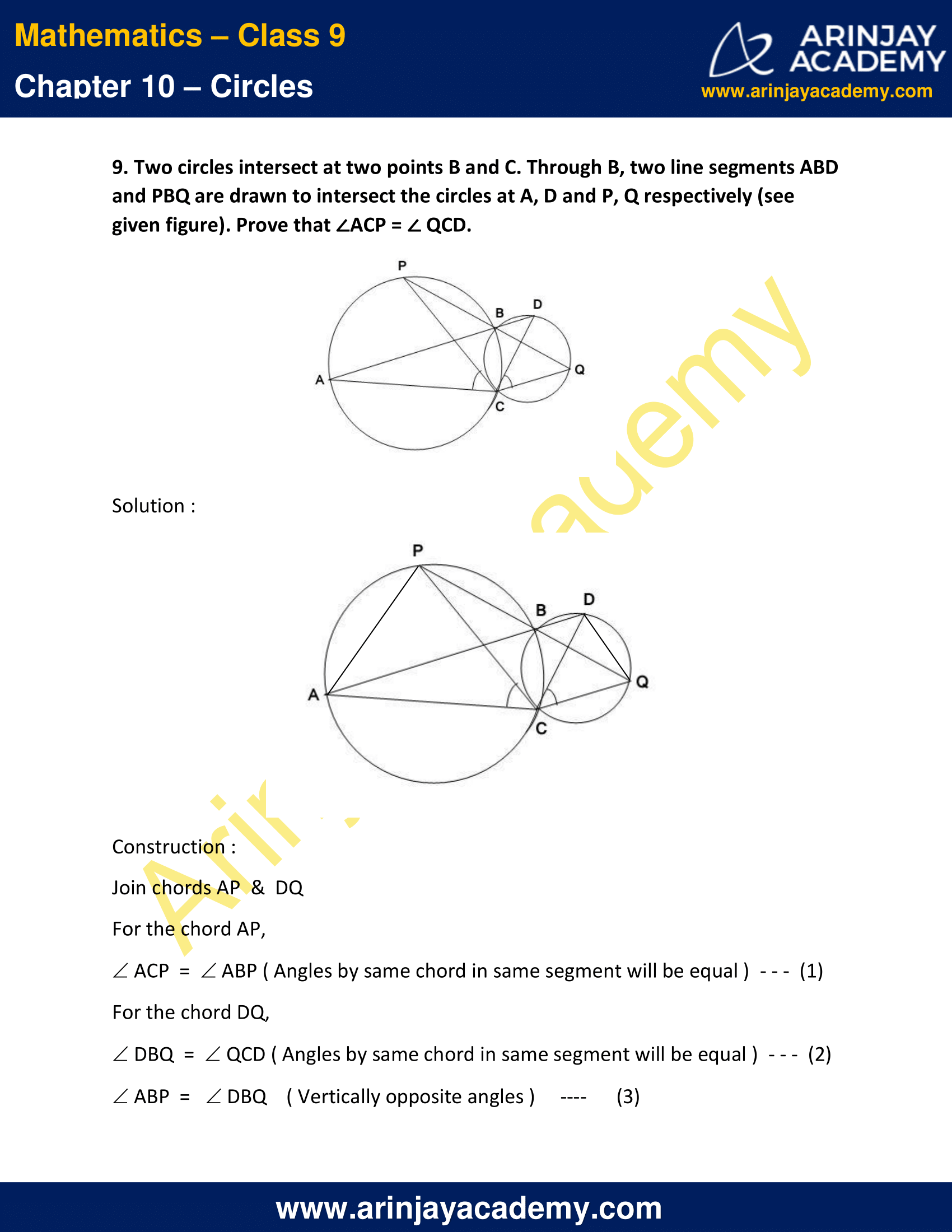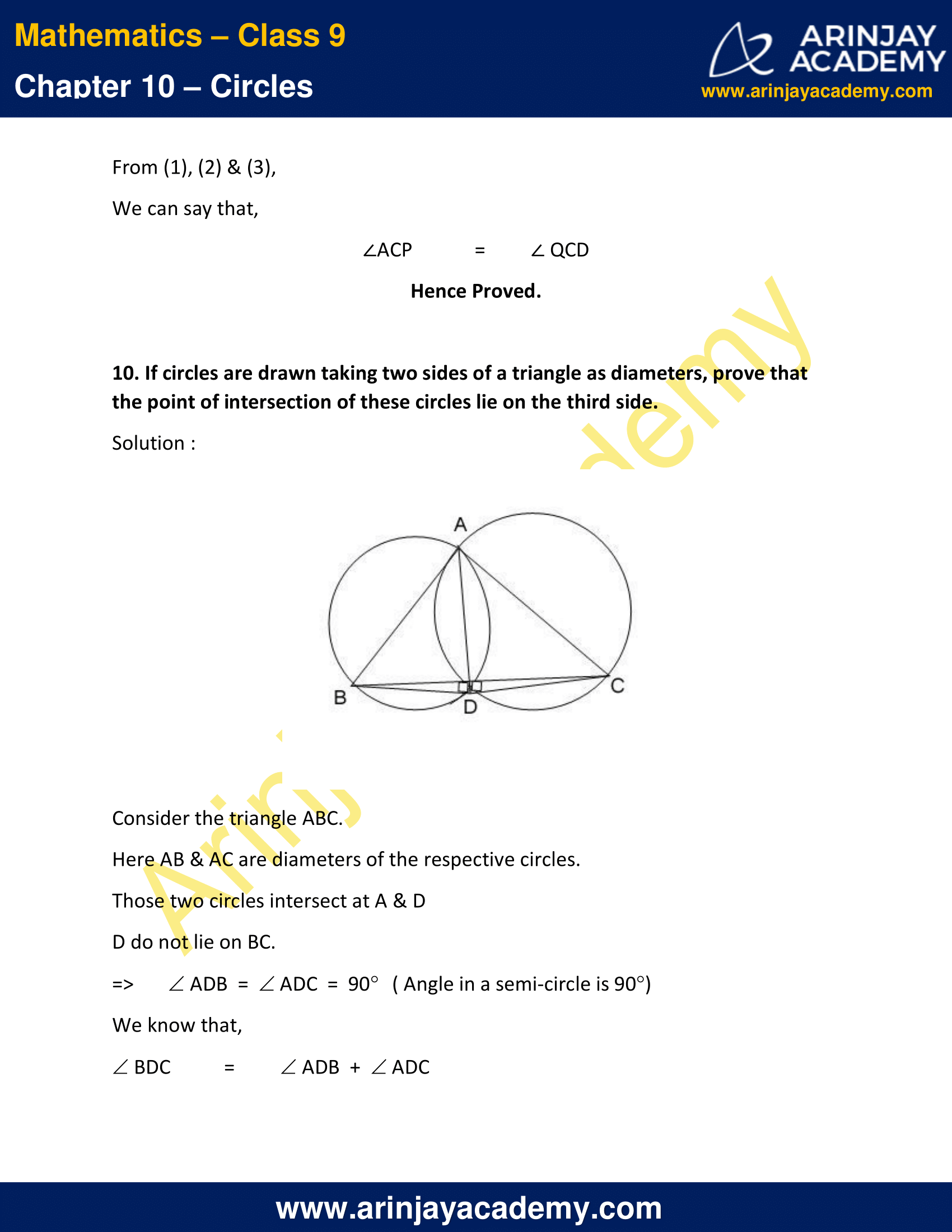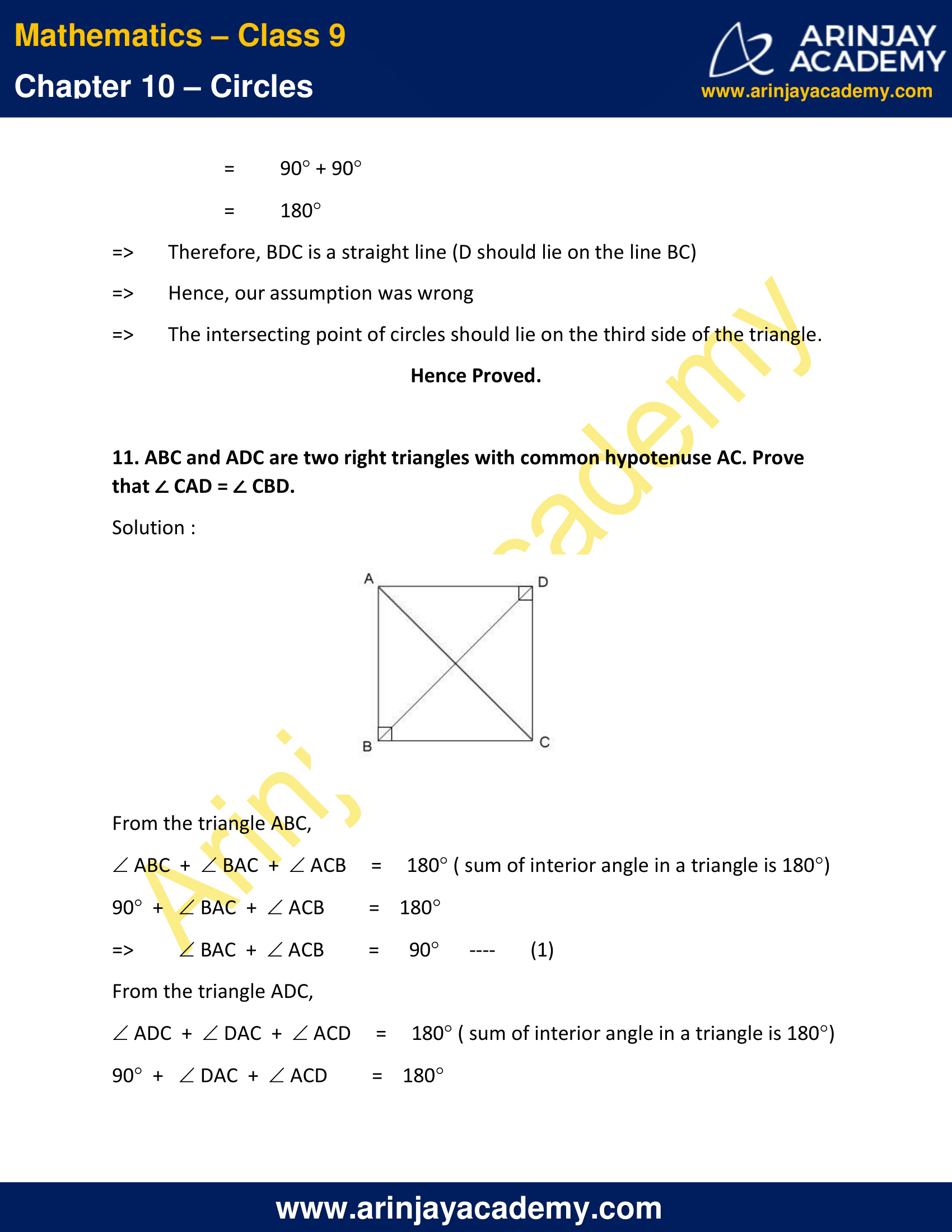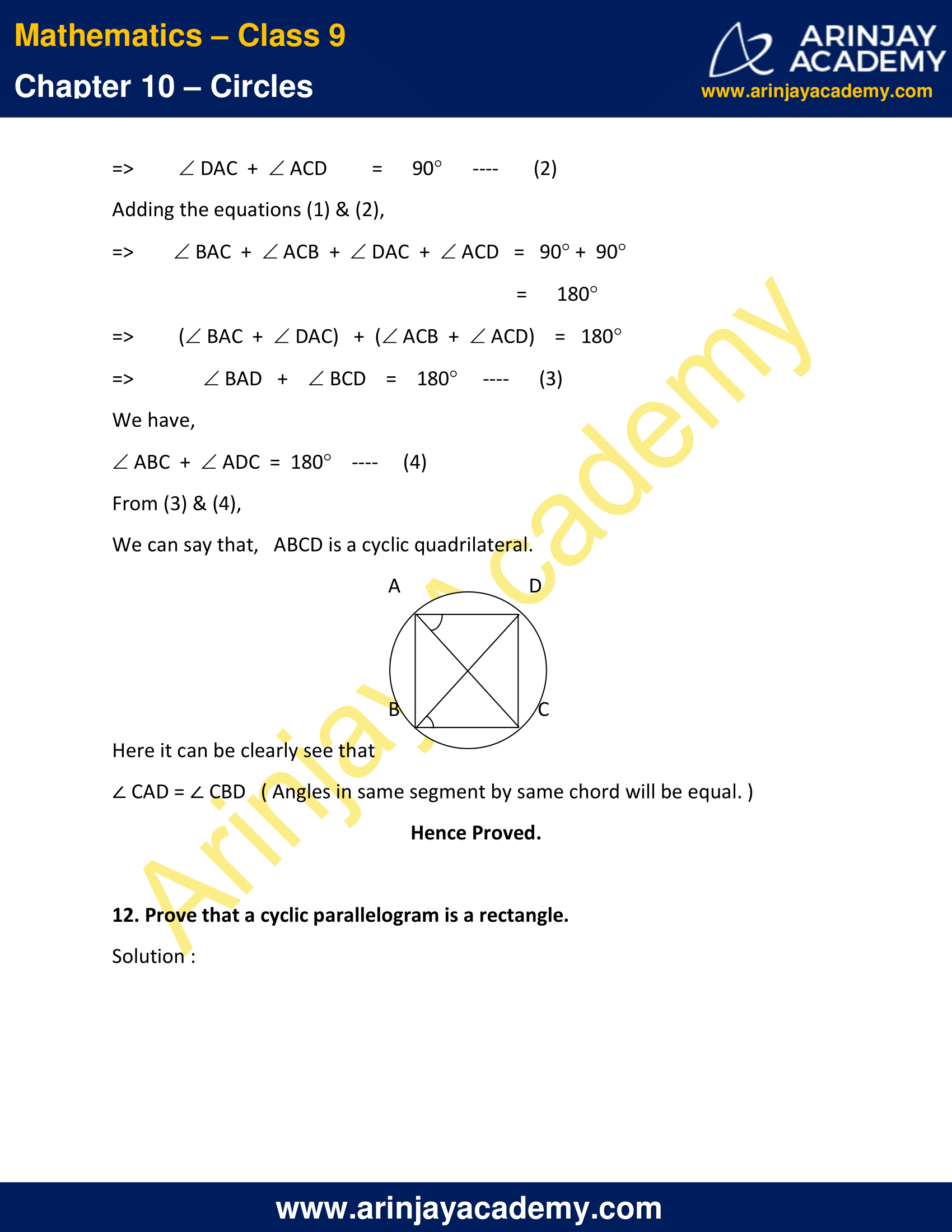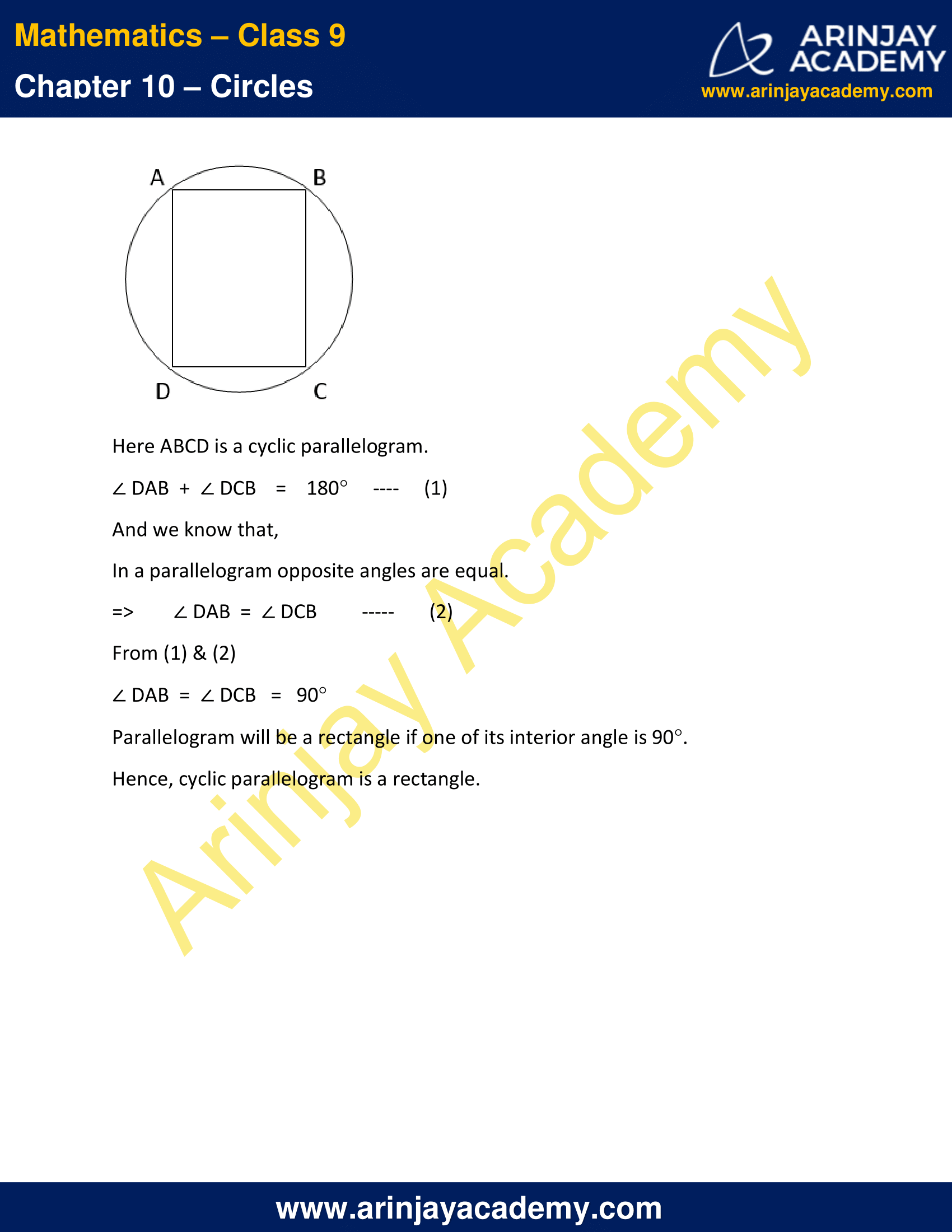NCERT Solutions for Class 9 Maths Chapter 10 Exercise 10.5 – Circles

1. In given figure, A,B and C are three points on a circle with centre O such that BOC = 30° and AOB = 60°. If D is a point on the circle other than the arc ABC, find ADC.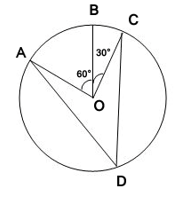Solution :

Here, it can be clearly observed that
∠AOC = ∠AOB + ∠BOC
= 60°  +  30°
= 90°
We know that,
The angle subtended by an arc at the centre is double the angle subtended by it any point on the remaining part of the circle.
=> ∠ADC = (1/2) ∠ AOC
=>  ∠ADC =  (1/2) x 90°
=>  ∠ADC = 45°

2. A chord of a circle is equal to the radius of the circle. Find the angle subtended by the chord at a point on the minor arc and also at a point on the major arc.

Solution :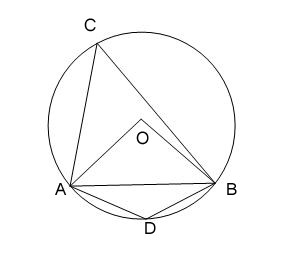In ∆ AOB,
AB  =  OA  =  OB  ( Given )
=> ∆ AOB is an equilateral triangle.
=> ∠ AOB = ∠ OAB = ∠ OBA = 60°
And that implies, ∠ ACB =  (1/2) ∠ AOB = 30°
Here, ADBC is a cyclic quadrilateral.
=> ∠ ACB  +  ∠ ADB = 180°
=> ∠ ADB = 180°  –  30°
=> ∠ ADB = 150°
Hence, the angle subtended by this chord at a point on the major arc and the minor arc are 30° and 150° respectively.

3. In given figure, PQR = 100°, where P, Q and R are points on a circle with centre O. Find OPR.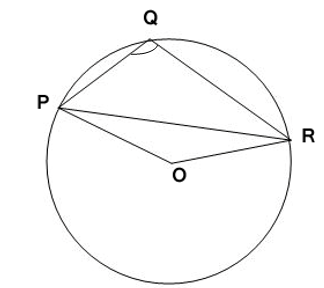Solution :

Given,  ∠ PQR = 100°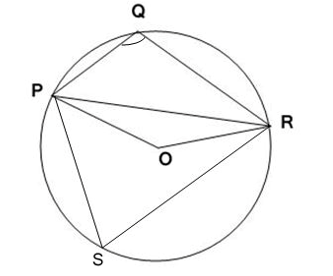Here, PR is the chord of the circle.
Let, S is any point on the major arc of the circle.
=> PQRS is a cyclic quadrilateral.
∠PQR  +  ∠ PSR = 180°
=> ∠ PSR = 180°  – 100°
=> ∠ PSR = 80°
We know that,
The angle subtended by an arc at the centre is double the angle subtended by it at any point on the remaining part of the circle.
=>  ∠ POR = 2 (∠ PSR )
= 2 x 80°
= 160°
In triangle POR,
OP  =  OR ( Both are Radii )
=> ∠ PRO  =  ∠ OPR
∠ POR  +  ∠ PRO  +  ∠ OPR = 180°
=> 2 ∠ OPR = 180°  –  160°
=> 2 ∠ OPR = 20°
=> ∠ OPR = 10°

4. In given figure, ABC = 69°, ACB = 31°, find BDC.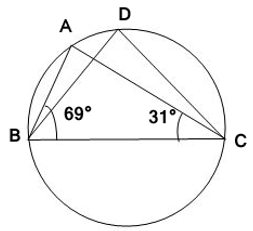Solution :

We know that,
Angles subtend by same chord in same segment will be equal.
=> ∠ BAC = ∠ BDC   ..(1)
In triangle ABC,
∠ BAC + ∠ ABC + ∠ ACB = 180°
=> ∠ BAC  +  69°  +  31° = 180°
=> ∠ BAC = 180°  –  100°
=> ∠ BAC = 80°
From (1)
∠ BDC =  80°

5. In given figure, A, B, C and D are four points on a circle. AC and BD intersect at a point E such that BEC = 130° and ECD = 20°. Find BAC.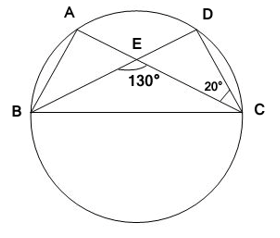Solution :

Since, BED is a straight line
∠ BEC  +  ∠ CED = 180°
=> ∠ CED = 180°  –  130°
=> ∠ CED = 50°
From the triangle EDC,
∠ EDC + ∠ CED + ∠ ECD = 180°
=> ∠ EDC = 180°  –  50°  –  20°
=> ∠ EDC = 110°
We know that,
∠ EDC = ∠ BDC
=> ∠ BDC = 110°
We know that,
Angles in same segment make by same chord will be equal.
=> ∠ BDC = ∠ BAC
=> ∠ BAC  = 110°

6. ABCD is a cyclic quadrilateral whose diagonals intersect at a point E. If DBC = 70°, BAC is 30°, find BCD. Further, if AB = BC, find ECD.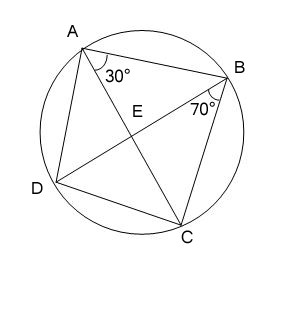Solution :

We know that,
Angles in the same segment form by same chord will be equal.
For chord CD,
∠CBD = ∠CAD
=> ∠CAD    =   70°
∠BAD = ∠CAD + ∠CAB
= 70°   +   30°
= 100°
Since ABCD is a cyclic quadrilateral,
∠BAD + ∠BCD = 180°
∠BCD = 180°  –  100°
∠BCD = 80°  ..(1)
In the triangle ABC,
AB  =  BC  ( given )
=> ∠BCA = ∠BAC
=  30°
∠BCD = ∠BCA + ∠ACD
=> ∠ ACD = 80°  –  30°
=> ∠ ACD = 50°
We know that,
∠ ACD = ∠ ECD
=> ∠ ECD = 50°

7. If diagonals of a cyclic quadrilateral are diameters of the circle through the vertices of the quadrilateral, prove that it is a rectangle.Solution :

Here, ABCD is a cyclic quadrilateral intersecting their diagonals AC & BD at center of the circle O.
Consider the chord BD,
=> ∠BAD = (1/2) ∠BOD
=> ∠BAD = (1/2) ( 180°)
=> ∠BAD = 90°
In a cyclic quadrilateral ABCD,
∠BAD  +  ∠BCD = 180°
=> ∠BCD = 180°  –  90°
=> ∠BCD = 90°
Consider the chord AC,
=> ∠ABC = (1/2) ∠AOC
=> ∠ABC = (1/2) ( 180°)
=> ∠ABC = 90°
In a cyclic quadrilateral ABCD,
∠ABC  +  ∠ADC = 180°
=> ∠ADC = 180°  –  90°
=> ∠ADC = 90°
Each interior angle of the cyclic quadrilateral is 90°.
=> Cyclic quadrilateral having diameters of the circle as its diagonals will be a rectangle.

Hence Proved.

8. If the non-parallel sides of a trapezium are equal, prove that it is cyclic.

Solution :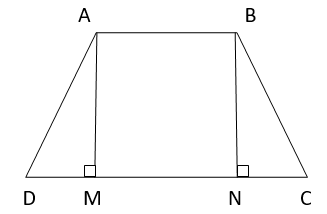Here, ABCD is a trapezium with AB || CD.
AD   =   BC  ( Given )
Construction :
Draw perpendiculars from A & B on CD.
=> AM  &  BN  are perpendicular to CD.
From the triangles AMD  &  BNC,
∠AMD = ∠BNC  (Each 90˚)
AM  =  BN  ( Perpendicular distance between parallel lines will be equal )
AD  =  BC  ( given )
=> ∆ AMD  &  ∆ BNC are congruent under R.H.S. congruency rule.
=> ∠ADC  =  ∠BCD  [By CPCT]  ..(1)
( Corresponding angles are equal in congruent triangles )
AB  || CD
AD is the transversal.
∠BAD  + ∠ADC = 180°
From  (1),
∠BAD  + ∠BCD = 180° ..(2)
∠ABC  +  ∠ADC  + ∠BAD  +  ∠BCD  = 360°
=> ∠ABC  +  ∠ADC = 180°   ..(3)
Equation (2) & Equation (3) shows that the opposite angles are supplementary.
We can say that,
If the non-parallel sides of a trapezium are equal, then it will be cyclic.
Hence Proved.

9. Two circles intersect at two points B and C. Through B, two line segments ABD and PBQ are drawn to intersect the circles at A, D and P, Q respectively (see given figure). Prove that ACP = QCD.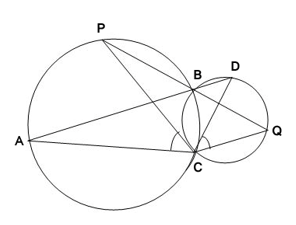Solution :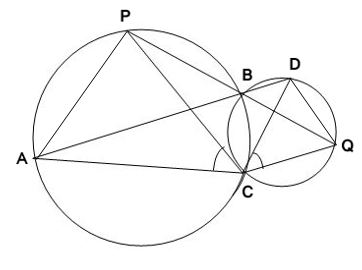Construction :
Join chords AP  &  DQ
For the chord AP,
∠ACP  =  ∠ABP ( Angles by same chord in same segment will be equal )  ..(1)
For the chord DQ,
∠DBQ  =  ∠QCD ( Angles by same chord in same segment will be equal )  ..(2)
∠ABP  =  ∠DBQ    ( Vertically opposite angles )  ..(3)
From (1), (2) & (3),
We can say that,
∠ACP = ∠ QCD
Hence Proved.

10. If circles are drawn taking two sides of a triangle as diameters, prove that the point of intersection of these circles lie on the third side.

Solution :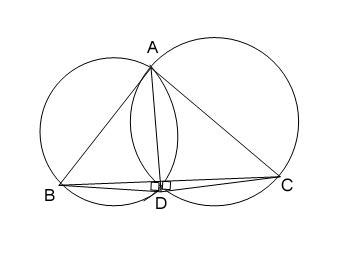Consider the triangle ABC.
Here AB & AC are diameters of the respective circles.
Those two circles intersect at A & D
D do not lie on BC.
=> ∠ADB  =  ∠ADC  =  90°   ( Angle in a semi-circle is 90°)
We know that,
∠BDC = ∠ADB  + ∠ADC
= 90° + 90°
= 180°
=> Therefore, BDC is a straight line (D should lie on the line BC)
=> Hence, our assumption was wrong
=> The intersecting point of circles should lie on the third side of the triangle.
Hence Proved.

11. ABC and ADC are two right triangles with common hypotenuse AC. Prove that CAD = CBD.

Solution :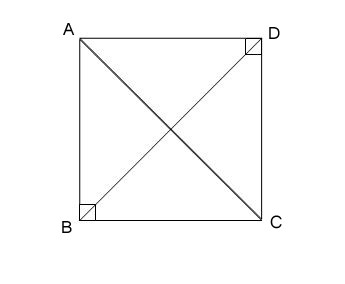From the triangle ABC,
∠ABC  +  ∠BAC  +  ∠ACB = 180° ( sum of interior angle in a triangle is 180°)
90°  +  ∠BAC  +  ∠ACB = 180°
=> ∠BAC  +  ∠ACB = 90°  ..(1)
From the triangle ADC,
∠ADC  +  ∠DAC  + ∠ACD = 180° ( sum of interior angle in a triangle is 180°)
90°  +  ∠DAC  +  ∠ACD = 180°
=> ∠DAC  + ∠ACD = 90°  ..(2)
Adding the equations (1) & (2),
=> ∠BAC  + ∠ACB  + ∠DAC  + ∠ACD = 90° +  90°
=  180°
=> (∠BAC  + ∠DAC)   +  (∠ACB  + ∠ACD) = 180°
=> ∠BAD +  ∠BCD = 180°  ..(3)
We have,
∠ABC  + ∠ADC  =  180° ..(4)
From (3) & (4),
We can say that, ABCD is a cyclic quadrilateral.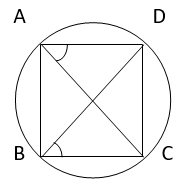Here it can be clearly see that
∠ CAD = ∠ CBD   ( Angles in same segment by same chord will be equal. )

Hence Proved.

12. Prove that a cyclic parallelogram is a rectangle.

Solution :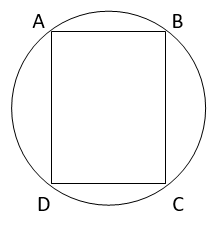Here ABCD is a cyclic parallelogram.
∠ DAB  +  ∠ DCB  = 180°   .. (1)
And we know that,
In a parallelogram opposite angles are equal.
=>∠ DAB  =  ∠ DCB   .. (2)
From (1) & (2)
∠ DAB  =  ∠ DCB   =   90°
Parallelogram will be a rectangle if one of its interior angle is 90°.
Hence, cyclic parallelogram is a rectangle.

NCERT Solutions for Class 9 Maths Chapter 10 Exercise 10.5 – Circles, has been designed by the NCERT to test the knowledge of the student on the following topics:-

• Angle Subtended by an Arc of a Circle
• Cyclic Quadrilaterals

Download NCERT Solutions for Class 9 Maths Chapter 10 Exercise 10.5 – Circles